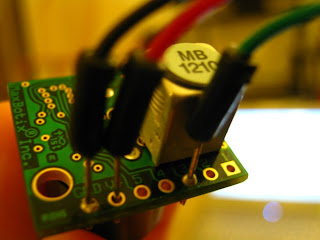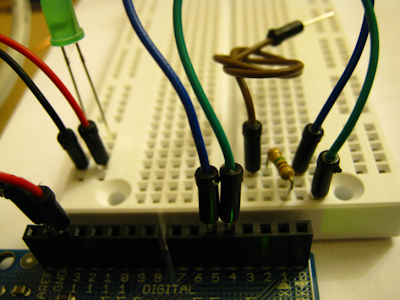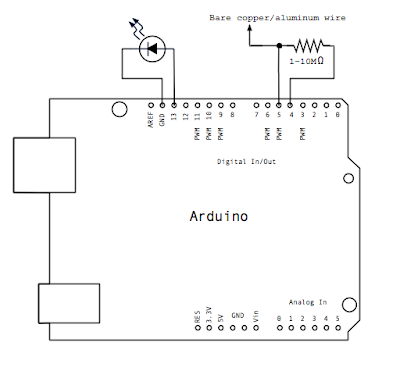### Fritzing.org: Create Awesome PCB layouts to document your projects

From the Fritzing website:

"We are creating a software and website in the spirit of Processing and Arduino, developing a tool that allows users to document their prototypes, sharethem with others, teach electronics in a classroom, and to create a pcb layout for professional manufacturing."

I'll be trying my hands on the Fritzing software next time I work on an Arduino project. Maybe one day I'll even have time to go back and fix my older posts to have better documentation à la Fritzing! :)

### Maxbotix XL-EZ2: Ultrasonic Range Finder

The Ultrasonic Range Finder is a sensor device that measures distance between the sensor and another object. It sends out an ultrasonic sound beam and measures the time taken for it to bounce back off of the closest object in front of it. Then depending on the length of the time interval, it determines the range (distance) to the nearest object in inches.
This kind of a sensor can be used in robotics to help navigate unmanned vehicles or in robotics to help them avoid running into walls or other objects. The rangefinder would detect when there is something in front of the vehicle and the vehicle or robot can be programmed to either stop or to try going around the obstacle. The ultrasonic rangefinders can also be used in stationary installations, like machines, appliances or artwork to activate certain switches based on the distance of a person from the sensor.

About the Maxbotix XL-EZ2 Ultrasonic Rangefinder:

The MaxSonar XL-EZ2 Ultrasonic Rangefinder by MaxBotix  used here gives the user an option of reading in the range either as serial, analog or PWM inputs.  The sample codes mentioned here use the analog input method (pin3 on the rangefinder) to read in the ranges. The ranges being read appear to be precise from around 20cm to up to even around five meters.  However the closer range values tended to fall into a dead zone on distances less than ~20cm when reading through the rangefinder's analog interface for the code below.It is also possible to read the data in PWM or serial format on this module. Separate pins are used for these (pin2 for PWM, pin5 for Serial data). The user can also set the Serial output pin's mode to read either asynchronous Serial data, or simply just a pulse instead.  The beam size of the rangefinder also varies depending upon the input voltage. It can be connected to 3.3V to up to 5V to increase total area that the ultrasonic beam covers. The code below can be used with either voltage.

Circuit:Schematic:Sample Arduino Program:
This code simply takes the input from the rangefinder's analog pin and checks it against a threshold to light up an LED if an object is too close.
 /*     This code takes in the input from the rangefinder's analog pin. The pin returns distance (in inches) from the sensor to the closest object in front of it. If an object is closer than the threshold distance, the LED lights up. Setup:Connect the analog pin (3/AX) of the rangefinder to the Arduino Board's Analog input pin0. Connect the ground and v++ on the rangefinder to a GND and 5V on the Arduino respectively. Connect the LED to pin13 and GND. By Naureen Mahmood */ #define THRESHOLD  30      // threshold distance in inches#define LED_PIN    13      // LED output pin#define RF_PIN      0      // Range Finder input pin void setup(){  Serial.begin(9600);  pinMode(RF_PIN, INPUT);    pinMode(LED_PIN, OUTPUT);  } void loop() {  // Read distance on Rangefinder's analog pin  int distance = analogRead(RF_PIN);  Serial.println(distance);          // Print measured distance    // if an object is closer than threshold distance, turn on LED  if (distance < THRESHOLD)    digitalWrite(LED_PIN, HIGH);  else     digitalWrite(LED_PIN, LOW);}

Sample Arduino Program (with averaged values to avoid jitters):
In this code, to avoid jitters, we take an average of 50 input samples before printing out the measured distance. If the measured distance is less than 30 inches, the LED on pin 13 is turned on.

 /*     This code takes in the input from the rangefinder's analog pin. The pin returns distance (in inches) from the sensor to the closest object in front of it. To avoid jitters, we take an average of 50 input samples before printing out the measured distance. If the measured distance is less than 30 inches, the LED on pin 13 is turned on. To avoid jitters, we take an average of 50 input samples before printing out the measured distance. If the measured distance is less than 30 inches, the LED on pin 13 is turned on. Setup:Connect the analog pin (AX/pin3) of the rangefinder to the Arduino Board's Analog input pin0. Connect the ground and v++ on the rangefinder to a GND and 5V on the Arduino respectively. By Naureen Mahmood */ #define THRESHOLD  40      // threshold distance in inches#define LED_PIN    13      // LED output pin#define RF_PIN      0      // Range Finder input pin int sampleSize   = 50;     // n readings 0 .. (n - 1)int sampleCount  = 0;      // to count how many samples taken so farint distance     = 0; void setup(){  Serial.begin(9600);  pinMode(RF_PIN, INPUT);    pinMode(LED_PIN, OUTPUT);  } void loop() {  // sampleCount starts life at 0, then loops through sampleSize  // at each iteration of this main loop    int avgDist;   distance += analogRead(RF_PIN);  sampleCount++;  if (sampleCount == sampleSize)   {    avgDist = distance/sampleSize;    Serial.println(avgDist);  //Print average of all measured values    sampleCount=0;      distance = 0;  }    // if an object is closer than threshold distance, turn on the LED  if (avgDist < THRESHOLD)    digitalWrite(LED_PIN, HIGH);  else     digitalWrite(LED_PIN, LOW);}

### DIY Touch Sensor (Capacitive Sensor)

Capacitive Sensors is a technology which detects proximity or touch (by a hand/skin, or any conductive object). The sensor measures the capacitance between the input and output nodes to detect a touch. The sensor detects anything that is conductive, so these sensors can be used to replace any normal switches to make them touch sensitive or even be utilized in making touch screens for monitors, touch-pads and touch sensitive buttons in phones, laptops or other devices.

About the Touch Sensor:
The sensor setup in the example below is a simple DIY setup without using a commercial sensor chip.

Setup:
Attach a high value resistor (1-10M Ohm) between an input and an output pin. Also connect a short bare copper or aluminum wire/foil to the input pin. If the wire is to be a longer one, make sure it isn't touching any other wires along the way, or just use a covered wire with a small uncovered area at its tip. This will be the touch sensor for the capacitive sensor (i.e. activates at touch).

An LED is also connected to a separate output pin and GND. This LED turns on when someone touches the sensor with a conductive object (e.g. capacitive sensors are most commonly used to sense touch with skin/fingers etc.)

It is also possible to vary the capacitance reading of this setup to detect even when one's hand is 3 to 4 inches from the sensor, or make it activate just on absolute touch. One can use lower values of R (e.g. 1 M Ohm or less) for absolute touch to activate the sensor. With a 10 M resistor the sensor should start to respond 1-2 inches away.

Code:
When the value at the output pin is changed from LOW to HIGH, it changes the state of input pin to LOW(or 0) for a very short time interval. This time interval is defined by:

T  =  R  x  C,

where
T  =  time interval,
R  =  resistance,
C  =  capacitance of the sensor + capacitance of any conductive object in contact with the sensor pin

So, this time interval increases if the sensor on input pin (the bare copper/aluminum wire) is touched with a conductive object. And the interval reduces again when the conductive
object is removed from the sensor.  So, we measure the length of the time interval to get a measure of capacitance on the touch sensor.

Threshold:
The value of the threshold here depends on how sensitive the user wants the sensor to be.  The lower bound of the threshold would be the value of R (the resistance) itself, since that remains constant in when measuring T = R x C. But, the upper bound can be changed depending on the requirements of the system.

Smoothing:
However, there might be a lot of jitter as well as environmental conditions that might make the
capacitance value jump around a lot. This can be overcome by using a smoothing function. For example, this can be done by reading the capacitance measure for a number of times and then averaging the values overall.

Circuit:Schematic:Sample Arduino Program:
When the output at pin4 transitions from LOW to HIGH, it changes the state of input pin5 to LOW(or 0) for a very short time interval. This time interval increases if the sensor on input pin5 is touched with a conductive object and vice versa.

At the start of each main loop cycle in this program, we set the value of a variable 'capX' to 0. Then for the time interval the value at input pin5 returns LOW, we increment 'capX'. This results in 'capX' being barely incremented if the sensor is not in contact with a conductive object. But, as soon as someone holds/touches the sensor the value of capX quickly increments because of the longer time interval. So, if the capX value is bigger than a given threshold, it means the sensor just detected a touch.

The value of the threshold here depends on how sensitive the user wants the sensor to be and/or the environmental affect the initial value at the sensor itself.

 /* This code turns the LED on while the sensor is in contact with a conductive material (e.g. when someone touches it with their bare skin/fingers) Setup:Attach a high value resistor (1-10M Ohm) between an output pin 4 and input pin 5. Also connect a short bare copper or aluminum wire/foil to the input pin5. Connect an LED to output pin13 and GND. By: Naureen Mahmood. */ #define LED        13#define THRESHOLD   5 int capI;      // interval when sensor pin 5 returns LOW void setup() {  Serial.begin(9600);  pinMode(LED, OUTPUT);  pinMode(4, OUTPUT);     // output pin  pinMode(5, INPUT);      // input pin} void loop() {  capI = 0;      // clear out capacitance measure at each loop   // transition output pin4 LOW-to-HIGH  to 'activate' sensor pin5  digitalWrite(4, HIGH);        // On activation, value of pin 5 stays LOW for a time interval T = R*C.   // C is big if the sensor is touched with a conductive object.  // Increment capI for the interval while pin5 is LOW  int val = digitalRead(5);  // read the input to be checked  while (val != HIGH){        capI++;        val = digitalRead(5);    // re-read the input to be checked   }  delay(1);    // transition output pin4 HIGH-to-LOW to 'deactivate' sensor pin5  digitalWrite(4, LOW);        Serial.println(capI, DEC);  // print out interval   if (capI > THRESHOLD)       // Turn LED on if capI is above threshold    digitalWrite(LED, HIGH);  else      digitalWrite(LED,  LOW);}

Sample Arduino Code (with smoothing filter):